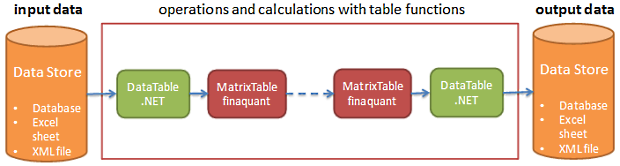# New release: finaquant® protos 1.02

### What is new in finaquant® protos release 1.02?

Subtable transformers and function routers are especially powerful constructs that enable the integration of table and matrix computations. These constructs can call matrix functions implemented with other numerical libraries like R, NLnumerics or matlab.

### What can you do with finaquant® protos 1.02?

Assume you are a student, or an academic staff, who is involved in some analytical projects that require historical data residing in some data tables as the primary input for all sort of computations like:

• Statistical estimations and forecasts
• Table mathematics
• Data preparation for reporting
• Scenario analysis
• Cause-effect networks and function trees with tables as input & output parameters

Following scenarios may give you an idea about the possible use of finaquant® protos provided that you have access to a computer with the .net framework of Microsoft installed on it.

### Integration of table data with matrix computations

By using high-level table functions like subtable transformers and function routers you can apply selected table functions on selected subtables (sub-data) of an input table.A DataTable object of the .net framework keeps table data in memory (RAM). You can read a table from a database into a DataTable object (using SQL-based commands) for further processing directly in the working memory.

MatrixTable class of finaquant is a mathematical representation of a table with a simplified data structure compared to DataTable. After you have converted a DataTable into a MatrixTable object with the corresponding function of the library, you can begin making operations and calculations with your tables just like you would do with matrices and vectors.

• Because you are making operations on in-memory data, your calculations will work possibly faster, and totally independent of the underlying data structure of the data store.
• You have a library of table functions that cover most of the required calculation patterns.

### Integration of table data with matrix computations

By using high-level table functions like subtable transformers and function routers you can apply selected table functions on selected subtables (sub-data) of an input table.You can replace table functions with matrix functions provided that all relevant parameters (i.e. subtable fields) are numbers. The matrix functions need not be written with the finaquant libraries only; they can be implemented with any library, including well-known software like R, ILNumerics and matlab.

### Filtering and sampling tables by dates

Assume, you have a data table containing daily prices of different products in each entry, and you want to exclude all rows of table associated with weekend days. That is, you want to have only entries with business days from Monday to Friday in your table. Then you need a date filter.

Assume, you have a data table containing temperatures measured at different locations almost daily (with some days missing), and you want to have only month-end measurements to calculate the yearly volatility of temperature for each location. If a measurement is missing on the last day of a certain month, the nearest previous temperature should be taken as a proxy value for the month-end day. In such a case, you need a date sampler with search options like previous (backward), next (forward) and nearest.

### Creating test tables

By using a table function like `CombinateTableRows` you can generate a test table with any number of dependent and independent attributes and random-valued key figures. This table function generates a table with all possible combinations of the rows of subtables you have given as input parameters.

This entry was posted in Calculation engine and tagged , , , . Bookmark the permalink.